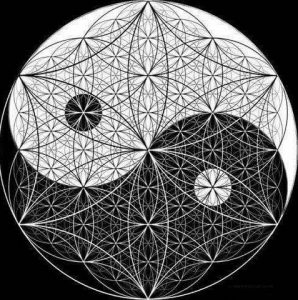# Counting in Geometry(a) In a plane, if there are n points of which no three are collinear, then

1. The number of straight lines that can be formed by joining them is nC2.
2. The number of triangles that can be formed by joining them is nC3.
3. The number of polygons with k sides that can be formed by joining them is nCk.

(b) In a plane, if there are n points out of which m points are collinear, then

1. The number of straight lines that can be formed by joining them is nC2mC2 + 1.
2. The number of triangles that can be formed by joining them is nC3mC3.
3. The number of polygons with k sides that can be formed by joining them is nCkmCk.

(c) The number of diagonals of an n sided polygon is nC2 – n = n × (n – 3)/2

(d) The number of triangles that can be formed by joining the vertices of an n-sided polygon which has,

1. Exactly one side common with that of the polygon is n × (n – 4).
2. Exactly two sides common with that of the polygon are n.
3. No side common with that of the polygon is n × (n – 4) × (n – 5)/6.

(e) The number of parallelograms formed if ‘x’ lines in a plane are intersected by ‘y’ parallel lines is x × y × (x – 1) × (y – 1)/4.

(f) If there are n lines drawn in a plane such that no two of them are parallel and no three of them are concurrent, then the number of different points at which these lines will intersect each other is nC2 = n × (n – 1)/2.

(g) If there are n straight lines in a plane with no two of them parallel to each other, no three passing through the same point and  their points of intersection are joined, then the number of new lines obtained are n × (n – 1) × (n – 2) × (n – 3)/8.

(h) The sides of a triangle a, b and c are integers where a ≤ b ≤ c. If c is given, then the number of different triangles is c × (c + 2)/4 or (c + 1)2/4, depending on whether c is even or odd. Also, the number of isosceles triangles is (3c – 2)/2 or (3c – 1)/2, depending on whether c is even or odd.

(i) In a square grid  of n × n,

1. The number of rectangles of any size is ∑r³.
2. The number of squares of any size is ∑r².

(j) In a rectangular grid  of p × q (p < q),

1. The number of rectangles of any size is p × q × (p + 1) × (q + 1) / 4.
2. The number of squares of any size is ∑ [(p + 1 – r) × (q + 1 – r)].

(k) If n straight lines are drawn in a plane such that no two lines are parallel and no three lines are concurrent, then the number of parts these lines divide the plane into is equal to [n × (n + 1)/2] + 1.

(l) If “n” parallel lines are passing through a circle, dividing the plane into distinct non-overlapping bounded or unbounded regions, then the maximum number of regions the plane can be divided into is (3n + 1).

## Solve the problem of the day

• Nice instruction, thanks

By LouisHayes , 3 months ago

• In a test match in cricket, the scores of Rohit and Vir...

By nhuadovan , 11 months ago

• The 111th digit of the series 149162536496481........ i...

By nhuadovan , 11 months ago

• how many perfect squares of natural numbers between 300...

By nhuadovan , 11 months ago

• Find the number of ways to distribute 2n different ball...

By nhuadovan , 11 months ago

• A cube is divided into two parts by a plane passing thr...

By nhuadovan , 11 months ago

• @utkarsh-garg25 how

By traciblack , 11 months ago

• Thank you for sharing the list ... i will try to read o...

By Anonymous , 1 year ago

• The collection of the questions are really great it wil...

By Anonymous , 2 years ago

• Mark bought 45 grinders for Rs. 2215, while carrying h...

By Anonymous , 2 years ago

• Great thread ,i tried to do these but not able to do..t...

By Anonymous , 2 years ago